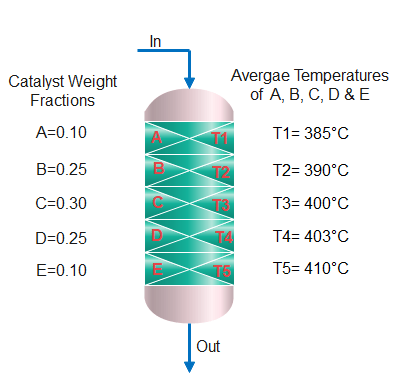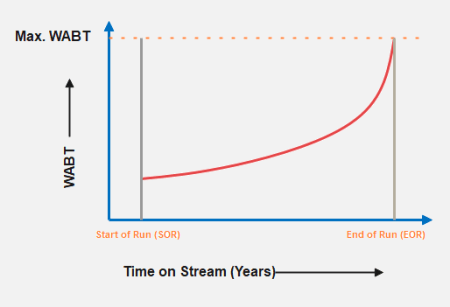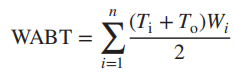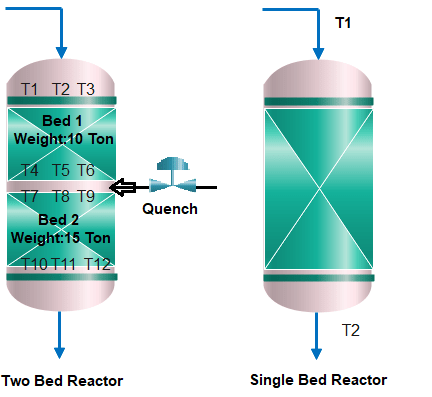# Weighted Average Bed Temperature Meaning, Significance and Measurement

The weighted average bed temperature or WABT describes the representative temperature of a catalytic reactor which has increasing or decreasing temperature from top to bottom of the reactor. The term “weighted” means that the average temperature is calculated based on the relative importance of each point on the bed, which is determined by the weight assigned to each point. The weight can be determined by the surface area or volume of each point, and it reflects the relative contribution of that point to the overall temperature of the bed.

WABT is used to indicate the relative activity of the catalyst, reaction rates monitoring and optimization, catalyst stability, catalyst life, and safe operation of the catalytic reactor. WABT is the key operating variable of the hydrotreating catalyst that helps to run the unit in an optimized way. WABT calculation provides a more accurate representation of the overall temperature of the bed, as it takes into account the temperature distribution across the bed.

The hydroprocessing reactions are exothermic, and the reactor outlet reactor temperature is higher than the inlet reactor temperature. This increase in reactor temperature from inlet to outlet is not linear. Therefore, an arithmetic average of the temperature of the reactor will not be the more accurate representative reactor temperature.

To properly monitor the reactor temperature, it is necessary to observe the temperature at the inlet and outlet and axially (and radially) throughout the catalyst bed. A temperature profile can be constructed by plotting the catalyst temperature versus the weight percent of the catalyst. For this reason, the weight average bed temperature (WABT) is typically used as a measure of the reactor temperature.#### Significance of Weighted Average Bed Temperature

The weighted average bed temperature of a hydrotreating catalyst is a significant parameter that is often used in the analysis and optimization of reactions. Here are some reasons why the weighted average bed temperature is important:

1. Reaction Rate: The rate of a catalytic reaction is highly dependent on the temperature of the catalyst bed. By calculating the weighted average bed temperature, you can estimate the reaction rate and optimize the temperature accordingly to achieve the desired reaction rates. With the increase in WABT the reaction rates will increase and vice versa.

Start of Run (SOR) conditions in the reactor are when the catalyst is freshly loaded and commissioned. At this stage, WABT is lowest during the catalyst life. The End of Run (EOR) stage is the condition when the catalyst is near to completing its life. At EOR temperatures thermal cracking becomes severe and can no longer be controlled by increasing Hydrogen partial pressure. Further, the coking rate on the catalyst bed also becomes excessive and the reactor’s differential pressure cal also becomes a limitation.1. Catalyst Stability: The stability of the catalyst is also dependent on the temperature of the bed. A high temperature may cause the catalyst to degrade or even lose its activity. Therefore, by monitoring the weighted average bed temperature, the catalyst’s stability can be ensured and optimized.
2. Energy Efficiency: The weighted average bed temperature can be used to determine the amount of energy required to maintain the desired temperature. By optimizing the temperature, the energy efficiency of the process can be improved, resulting in cost savings and environmental benefits.
3. Safety: Catalytic hydrotreating/hydrocracking reactions can be highly exothermic, and the temperature of the catalyst bed must be carefully controlled to avoid runaway reactions and safety hazards. The weighted average bed temperature can be used to monitor and control the temperature to ensure safe operation. Hydrocracking Unit designs include a safety feature that depressurizes the hydrocracking reactor whenever a bed temperature gets too high using an emergency depressurization system. The quick drop in pressure will alleviate the chance of a runaway chain reaction.
4. Relative Activity of Catalyst: The WABT is used to indicate the catalyst activity in catalytic processes, where deactivation of the catalyst starts to take place from the start-up after a catalyst change. To maintain the catalyst activity despite the deactivation, the inlet temperature is gradually increased by the operators and the temperature profile through the reactor changes over time. WABT at the start of a run is compared to WABT at a given time after start-up, and the difference is telling something about the degree of catalyst deactivation.#### Difference Between Peak and WABT Temperature

Peak temperature shows the maximum temperature at any location of the catalyst bed while WABT is the average temperature of the complete catalyst in the reactor. The peak temperature is important from the safety point of view and helps to avoid maximum temperature and temperature runaway of the catalyst. WABT is important for the optimization and activity aspect of the catalyst.

#### Calculating Weight Average Bed Temperature

There are two basic methods by which the WABT is determined: Method 1 can be stated simply as follows:where;

• n=number of reactor beds
• Ti =reactor bed inlet temperature
• To =reactor bed outlet temperature
• Wi = weight fraction of total catalyst in reactor bed i

An alternative method 2 is as follows;This second method takes into account the fact that some hydroprocessing reactions are nonlinear resulting in a somewhat rapid initial temperature rise followed by a more gradual temperature rise near the end of the catalyst bed. The particular method to be used may be customary to a given catalyst vendor or catalyst type, and it is important to determine which method to use so that the catalyst performance can be properly judged.

#### WABT Calculation Example 1: One Catalyst Bed With Two Temperature IndicatorsIn this case, both N and Wci are 1, and Tin = T1 and Tout = T2, using method no 2 described above;

WABT = (Tin + 2*Tout)/3 * 1

If T1=325 °C, T2=380°C,

WABT = (325°C + 2*380°C)/3 = 362°C

#### WABT Calculation: Two Catalyst Beds With Multiple Temperature Indicators

Using Method 1; In this case;

• n = number of reactor beds is 2,
• Total Catalyst Weight = 25 Ton,
• Wf Bed1= weight fraction of Bed 1 = 0.4 , W2= Wight Fraction of Bed 2= 0.6
• T1= 325°C, T2= 327°C, T3= 328°C, T4 = 350°C, T5= 348°C, T6=353°C, T7= 345°C, T8= 344°C, T9= 346°C, T10=360°C, T11=362°C, T12= 359°C

Bed 1 T in= 326.6 °C (Avg. of T1, T2 & T3), Bed 1 T out = 350.3 °C (Avg. of T4, T5 & T6)

Bed 2 T in = 345°C (Avg. of T7, T8 & T9), Bed 2 T out = 360.3 °C(Avg. of T10, T11 & T12)

WABT= (326.6+350.3)/2*0.4+ (345+360.3)/2*0.6

WABT= 135.38+ 211.59= 347 °C.

Using Method 2;

WABT Calculations Examples

In this case, there are three indications at each height level in the reactor, and the Tin and Tout of each fraction is the average of these three indications.

Bed 1 T in= 326.6 °C (Avg. of T1, T2 & T3), Bed 1 T out = 350.3 °C (Avg. of T4, T5 & T6)

Bed 2 T in = 345°C (Avg. of T7, T8 & T9), Bed 2 T out = 360.3 °C(Avg. of T10, T11 & T12)

WABT = (Tin + 2*Tout)/3 * Wf

WABT Bed 1= (326.6+2*350.3)/3*0.4 = 136.96

WABT Bed 2=(345+2*360.3)/3*0.6= 213.12

WABT = WABT Bed 1+ WABT Bed 2 = 136.96+213.12 = 350 °C

#### Top References

1. Deactivation of Heavy Hydroprocessing Catalysts by JORGE ANCHEYTA.
2. Hydroprocessing for Clean Energy by FRANK (XIN X.) ZHU, RICHARD HOEHN, VASANT THAKKAR, EDWIN YUH
3. The Engineering Toolbox
Previous Article